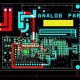# Best Interview Questions and Answer about Electrical Transformer

In this tutorial we are going to learn about Best Interview Questions and Answer about Electrical Transformer.

Question1. Which form a transformer transform?

Answer – A transformer transform in from of voltage.

Question2. How many types of transformer?

Answer – Two types of transformer are there like one step up and second is step down.

Question3. Which of the current, voltage and frequency does not change in transformer?

Answer – Frequency can’t change in transformer.

Question4. How the energy is conveyed from primary to secondary in transformer?

Answer – The energy is conveyed from primary to secondary in transformer by the Flux.

Question5. Which winding has more number of turn in transformer?

Answer – High Voltage winding has more number of turn in transformer.

Question6. What is method of cooling a power transformer?

Answer – Oil cooling is method of a power transformer.

Question7. What is constant in power transformer?

Answer – Main flux always constant in power transformer.

Question8. Where the tapping used in transformer?

Answer – Low Voltage side the tapping used in transformer.

Question9. Which is rating form of transformer?

Answer – KVA is rating form of transformer.

Question10. Which material use for transformer core?

Answer – Silicon Steel use for transformer core.

Question11. What is thickness of lamination used in transformer?

Answer – 0.4mm to 0.55 is thickness of lamination used in transformer.

Question12. What is resistance between primary winding and secondary winding in transformer?

Answer – Infinite resistance between primary winding and secondary winding in transformer.

Question13. When the maximum efficiency of a distribution transformer?

Answer – At 50% full load is the maximum efficiency of a distribution transformer.

Question14. Why use silicon steel in lamination of transformer?

Answer – Hysteresis losses

Question15. What is ideal Transformer?

Answer – No losses and magnetic leakage.

Question16. How to measure iron loss of a transformer?

Answer – By low power factor wattmeter use to measure iron loss of a transformer.

Question17. What is form of noise in transformer?

Answer – Humming is form of noise in transformer.

Question18. Why do use KVA in transformer?

Answer – Because total transformer loss depends on volt-ampere.

Question19. When the ideal transformers have maximum efficiency at load?

Answer – When the copper loss = iron loss

Question20. How to test of open circuit for transformer?

Answer – When the primary is supplied rated voltage.## About EEE

We have designing Experience for the last 40 years.